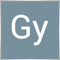160

Hi programmers,
Hope you are having good days.

I have a problem which I can not find my mistake, could you please point me the problem with this?
It would be greatly appreciated if you give me a hand.

```#include "TicksInfo.mqh"
CTicksInfo ticksInfo;

string AllPairs[];

int tickCount = 0;

////////////////////

int OnInit()
{

/*
* Test Printout
*/
for (int i=0; i<ArraySize(AllPairs); i++)
Print ("Currency Pair ", i, " is ", AllPairs[i]);

ticksInfo.init(AllPairs);

return(INIT_SUCCEEDED);
}

/////////////////

void OnDeinit(const int reason)
{
ticksInfo.deInit();
Print ("Total Ticks = ", tickCount);
}

///////////////

void OnTick()
{
bid=Bid;

tickCount++;
//Print ("=== Now process tick ", tickCount);
MqlTick myTick;

if (ticksInfo.getTick(_Symbol,myTick)==false)
//printTick (_Symbol,myTick);
//else
Print (_Symbol, " Error");

for(int i=1; i<ArraySize(AllPairs); i++)
{
if (ticksInfo.getTick(AllPairs[i],myTick))
{
//printTick (AllPairs[i],myTick);
bid[i] = myTick.bid;
}
else
Print (AllPairs[i], " Error");
}
Comment(ask, " , ", ask, " , ", ask, "\n", bid, " , ", bid, " , ", bid);

if(Hour()==6)ExpertRemove();
}

///////////////////

/* GLOBAL AREA*/

void printTicktoFile(string sym, MqlTick &tick)
{
FileSeek(file,0,SEEK_END);
FileWrite(file, sym, " tick number ", tickCount, ", at ",
tick.time, " ",
IntegerToString(tick.time_msc-(tick.time*1000),3,'0'),
FileClose(file);
}

void printTick(string sym, MqlTick &tick)
{
Print (sym, " tick number ", tickCount, ", at ",
tick.time, " ",
IntegerToString(tick.time_msc-(tick.time*1000),3,'0'),
}```

I store Ask/Bid price of each tick for other currencies. Then I run my EA which process based on multi-currencies.
THE PROBLEM is: I only receive the starting price of my ask/bid, ask/bid etc. In other words it only catches the price of other currencies at the beginning of date of EA I do backtest and not ask/bid after the first tick of absent currencies.
where should be fixed?14

HosseinKOGO:

I store Ask/Bid price of each tick for other currencies. Then I run my EA which process based on multi-currencies.

THE PROBLEM is: I only receive the starting price of my ask/bid, ask/bid etc. In other words it only catches the price of other currencies at the beginning of date of EA I do backtest and not ask/bid after the first tick of absent currencies.
where should be fixed?

You cannot do multicurrency backtests in MT4.